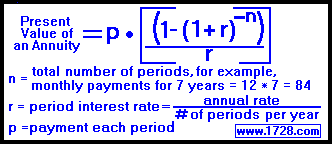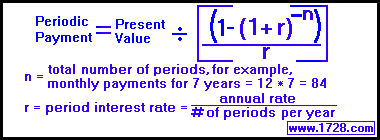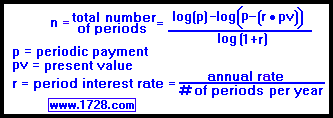Formulas for Calculating
The Present Value of an Ordinary Annuity

 1) Solving the Present ValueA friend offers to buy your car if he can pay you \$100 per month for 3 years at an annual interest rate of 7.5% What is the present value of all these payments? The payment per period ('p') is \$100 the total number of periods ('n') is: 12 periods per year for 3 years, equals 12*3 = 36 the interest rate is .075 ÷ 12 = 0.00625 Putting these numbers into the formula: Present Value = 100 * [(1-(1.00625)^-36)/.00625] Present Value = 100 * [(1-0.799075542783109)/.00625] Present Value = 100 * [(0.200924457216891)/.00625] Present Value = 100 * 32.1479131547025 Present Value = 3,214.79

 2) Solving the Periodic Payment(Using the data from question 1) A 3 year 7.5% monthly annuity has a present value of \$3,214.79. What was the periodic payment? The total number of periods ('n') is: 12 periods per year for 3 years, equals 12*3 = 36 the interest rate is .075 ÷ 12 = 0.00625 the present value is \$3,214.79 Putting these numbers into the formula: Payment= 3,214.79 ÷ [(1-(1.00625)^-36) / 0.00625] Payment= 3,214.79 ÷ ((1-0.799075542783109) / 0.00625) Payment= 3,214.79 ÷ (0.200924457216891 / 0.00625) Payment= 3,214.79 ÷ 32.1479 Payment= 100.00

 3) Solving for Years(Using the data from question 1) A 7.5% annuity with \$100 monthly payments has a present value of \$3,214.79 How many years is this annuity for? the interest rate is .075 ÷ 12 = 0.00625 the present value is \$3,214.79 the monthly payment =100 Putting these numbers into the formula: n=[Log(100) ― Log((100 -(0.00625)*3,214.79))] / Log(1.00625) n=[Log(100) ― Log((100 -(20.0924375)))] / Log(1.00625) n=[Log(100) ― Log((79.9075625))] / Log(1.00625) n=(2 -1.9025878832)/ 0.00270589337592 n=0.0974121168 / 0.00270589337592 n=35.9999834682621 n=36 total number of periods This is a monthly annuity so, dividing 36 by 12 equals 3 years.

 4) Solving for Rate The present value of an ordinary annuity formula cannot be solved for rate. The present value calculator solves for rate by using a trial and error process.

RETURN   TO   HOME   PAGE